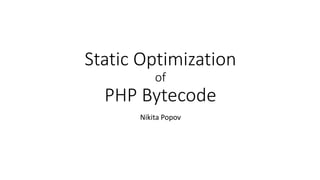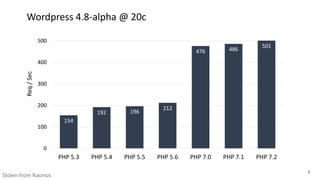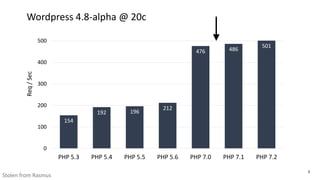Successfully reported this slideshow.

# Static Optimization of PHP bytecode (PHPSC 2017)

12

Share×
1 of 74
1 of 74

# Static Optimization of PHP bytecode (PHPSC 2017)

12

Share

Introduction to SSA-based opcode optimizations for PHP.

Introduction to SSA-based opcode optimizations for PHP.

## More Related Content

### Related Books

Free with a 30 day trial from Scribd

See all

### Related Audiobooks

Free with a 30 day trial from Scribd

See all

### Static Optimization of PHP bytecode (PHPSC 2017)

1. 1. Static Optimization of PHP Bytecode Nikita Popov
2. 2. 2 154 192 196 212 476 486 501 0 100 200 300 400 500 PHP 5.3 PHP 5.4 PHP 5.5 PHP 5.6 PHP 7.0 PHP 7.1 PHP 7.2 Req/Sec Stolen from Rasmus Wordpress 4.8-alpha @ 20c
3. 3. 3 154 192 196 212 476 486 501 0 100 200 300 400 500 PHP 5.3 PHP 5.4 PHP 5.5 PHP 5.6 PHP 7.0 PHP 7.1 PHP 7.2 Req/Sec Stolen from Rasmus Wordpress 4.8-alpha @ 20c
4. 4. 4 \$a = 42; \$b = 24; echo \$a + \$b; Code
5. 5. 5 ASSIGN \$a, 42 ASSIGN \$b, 24 T0 = ADD \$a, \$b ECHO T0 \$a = 42; \$b = 24; echo \$a + \$b; Compile Code Opcodes
6. 6. 6 ASSIGN \$a, 42 ASSIGN \$b, 24 T0 = ADD \$a, \$b ECHO T0 \$a = 42; \$b = 24; echo \$a + \$b; Compile Virtual Machine Execute Code Opcodes
7. 7. 7 ASSIGN \$a, 42 ASSIGN \$b, 24 T0 = ADD \$a, \$b ECHO T0 \$a = 42; \$b = 24; echo \$a + \$b; Compile Virtual Machine Execute Code Opcodes Optimize
8. 8. 8 ASSIGN \$a, 42 ASSIGN \$b, 24 T0 = ADD \$a, \$b ECHO T0 \$a = 42; \$b = 24; echo \$a + \$b; Compile Virtual Machine Execute Code Opcodes Optimize SSA
9. 9. 9 Optimizations
10. 10. 10 \$c = \$a + \$b; T0 = ADD \$a, \$b ASSIGN \$c, T0 Optimizations Specialization
11. 11. 11 \$c = \$a + \$b; T0 = ADD \$a, \$b ASSIGN \$c, T0 \$c = ADD \$a, \$b Optimizations Specialization
12. 12. 12 \$c = \$a + \$b; T0 = ADD \$a, \$b ASSIGN \$c, T0 \$c = ADD \$a, \$b \$c = ADD_INT \$a, \$b Optimizations Specialization
13. 13. 13 \$c = \$a + \$b; T0 = ADD \$a, \$b ASSIGN \$c, T0 \$c = ADD \$a, \$b \$c = ADD_INT \$a, \$b \$c = ADD_INT_NO_OVERFLOW \$a, \$b Optimizations Specialization
14. 14. 14 Optimizations Constant Propagation \$a = 2; \$b = \$a + 1; echo \$a * \$b;
15. 15. 15 Optimizations Constant Propagation \$a = 2; \$b = 3; echo 6;
16. 16. 16 Optimizations Constant Propagation & Dead Code Elimination echo 6;
17. 17. 17 Optimizations Inlining
18. 18. 18 Optimizations Inlining function test() { var_dump(div(4, 2)); } function div(\$a, \$b) { if (\$b == 0) { return -1; } return \$a / \$b; }
19. 19. 19 Optimizations Inlining function test() { var_dump(div(4, 2)); } function div(\$a, \$b) { if (\$b == 0) { return -1; } return \$a / \$b; } function test() { \$a = 4; \$b = 2; if (\$b == 0) { \$retval = -1; goto end; } \$retval = \$a / \$b; goto end; end: unset(\$a, \$b); var_dump(\$retval); }
20. 20. 20 Optimizations Inlining + CP function test() { var_dump(div(4, 2)); } function div(\$a, \$b) { if (\$b == 0) { return -1; } return \$a / \$b; } function test() { \$a = 4; \$b = 2; \$retval = 2; goto end; end: unset(\$a, \$b); var_dump(2); }
21. 21. 21 Optimizations Inlining + CP + DCE function test() { var_dump(div(4, 2)); } function div(\$a, \$b) { if (\$b == 0) { return -1; } return \$a / \$b; } function test() { var_dump(2); }
22. 22. 22 SSA Form and Type Inference
23. 23. 23 \$x = 42; if (cond) { \$x = 42.0; var_dump(\$x); } else { \$x = "42"; var_dump(\$x); } var_dump(\$x); Static Single Assignment (SSA) Form
24. 24. 24 \$x = 42; if (cond) { \$x = 42.0; var_dump(\$x); } else { \$x = "42"; var_dump(\$x); } var_dump(\$x); Static Single Assignment (SSA) Form Type of \$x: int|float|string
25. 25. 25 \$x = 42; if (cond) { \$x = 42.0; var_dump(\$x); } else { \$x = "42"; var_dump(\$x); } var_dump(\$x); Static Single Assignment (SSA) Form Type of \$x here: int Type of \$x here: float Type of \$x here: string Type of \$x here: float|string
26. 26. 26 \$x = 42; if (cond) { \$x = 42.0; var_dump(\$x); } else { \$x = "42"; var_dump(\$x); } var_dump(\$x); Static Single Assignment (SSA) Form
27. 27. 27 Static Single Assignment (SSA) Form \$x_1 = 42; if (cond) { \$x_2 = 42.0; var_dump(\$x_2); } else { \$x_3 = "42"; var_dump(\$x_3); } var_dump(\$x_?);
28. 28. 28 Static Single Assignment (SSA) Form \$x_1 = 42; if (cond) { \$x_2 = 42.0; var_dump(\$x_2); } else { \$x_3 = "42"; var_dump(\$x_3); } \$x_4 = phi(\$x_2, \$x_3); var_dump(\$x_4);
29. 29. 29 Static Single Assignment (SSA) Form \$x_1 : int \$x_2 : float \$x_3 : string \$x_4 : float|string \$x_1 = 42; if (cond) { \$x_2 = 42.0; var_dump(\$x_2); } else { \$x_3 = "42"; var_dump(\$x_3); } \$x_4 = phi(\$x_2, \$x_3); var_dump(\$x_4);
30. 30. 30 Type Inference \$x = 42; do { \$y = \$x; \$x = \$x + 3.14; } while (cond); var_dump(\$y);
31. 31. 31 Type Inference \$x_1 = 42; do { \$x_2 = phi(\$x_1, \$x_3); \$y_1 = \$x_2; \$x_3 = \$x_2 + 3.14; } while (cond); var_dump(\$y_1);
32. 32. 32 Type Inference \$x_1 = 42; do { \$x_2 = phi(\$x_1, \$x_3); \$y_1 = \$x_2; \$x_3 = \$x_2 + 3.14; } while (cond); var_dump(\$y_1);
33. 33. 33 Type Inference \$x_1 = 42; do { \$x_2 = phi(\$x_1, \$x_3); \$y_1 = \$x_2; \$x_3 = \$x_2 + 3.14; } while (cond); var_dump(\$y_1); \$x_1 : int \$x_2 : ∅ \$y_1 : ∅ \$x_3 : ∅
34. 34. 34 Type Inference \$x_1 = 42; do { \$x_2 = phi(\$x_1, \$x_3); \$y_1 = \$x_2; \$x_3 = \$x_2 + 3.14; } while (cond); var_dump(\$y_1); \$x_1 : int \$x_2 : ∅ \$y_1 : ∅ \$x_3 : ∅
35. 35. 35 Type Inference \$x_1 = 42; do { \$x_2 = phi(\$x_1, \$x_3); \$y_1 = \$x_2; \$x_3 = \$x_2 + 3.14; } while (cond); var_dump(\$y_1); \$x_1 : int \$x_2 : int \$y_1 : ∅ \$x_3 : ∅
36. 36. 36 Type Inference \$x_1 = 42; do { \$x_2 = phi(\$x_1, \$x_3); \$y_1 = \$x_2; \$x_3 = \$x_2 + 3.14; } while (cond); var_dump(\$y_1); \$x_1 : int \$x_2 : int \$y_1 : ∅ \$x_3 : ∅
37. 37. 37 Type Inference \$x_1 = 42; do { \$x_2 = phi(\$x_1, \$x_3); \$y_1 = \$x_2; \$x_3 = \$x_2 + 3.14; } while (cond); var_dump(\$y_1); \$x_1 : int \$x_2 : int \$y_1 : int \$x_3 : float
38. 38. 38 Type Inference \$x_1 = 42; do { \$x_2 = phi(\$x_1, \$x_3); \$y_1 = \$x_2; \$x_3 = \$x_2 + 3.14; } while (cond); var_dump(\$y_1); \$x_1 : int \$x_2 : int \$y_1 : int \$x_3 : float
39. 39. 39 Type Inference \$x_1 = 42; do { \$x_2 = phi(\$x_1, \$x_3); \$y_1 = \$x_2; \$x_3 = \$x_2 + 3.14; } while (cond); var_dump(\$y_1); \$x_1 : int \$x_2 : int|float \$y_1 : int \$x_3 : float
40. 40. 40 Type Inference \$x_1 = 42; do { \$x_2 = phi(\$x_1, \$x_3); \$y_1 = \$x_2; \$x_3 = \$x_2 + 3.14; } while (cond); var_dump(\$y_1); \$x_1 : int \$x_2 : int|float \$y_1 : int \$x_3 : float
41. 41. 41 Type Inference \$x_1 = 42; do { \$x_2 = phi(\$x_1, \$x_3); \$y_1 = \$x_2; \$x_3 = \$x_2 + 3.14; } while (cond); var_dump(\$y_1); \$x_1 : int \$x_2 : int|float \$y_1 : int|float \$x_3 : float
42. 42. 42 Type Inference \$a = 2**62; \$b = 2**62; var_dump(\$a + \$b); // float(9.2233720368548E+18)
43. 43. 43 Type Inference \$a = 2**62; \$b = 2**62; var_dump(\$a + \$b); // float(9.2233720368548E+18) Accurate type inference requires value range inference!
44. 44. 44 Type Inference \$a = 2**62; \$b = 2**62; var_dump(\$a + \$b); // float(9.2233720368548E+18) Accurate type inference requires value range inference! Same basic concept as type inference, but technically more involved…
45. 45. 45 Optimization obstacles
46. 46. 46 eval()? variable variables?
47. 47. 47 eval()? variable variables? Don't optimize functions using those!
48. 48. 48 function test() { \$foobar = 42; func(\$foobar); var_dump(\$foobar); // int(42)? } References
49. 49. function func(&\$ref) { \$ref = 24; } function test() { \$foobar = 42; func(\$foobar); var_dump(\$foobar); // int(42)? nope! } 49 References
50. 50. // file1.php function func(&\$ref) { \$ref = 24; } // file2.php function test() { \$foobar = 42; func(\$foobar); var_dump(\$foobar); // int(42)? nope! } 50 References
51. 51. 51 References Files compiled independently // file1.php function func(&\$ref) { \$ref = 24; } // file2.php function test() { \$foobar = 42; func(\$foobar); var_dump(\$foobar); // int(42)? nope! }
52. 52. 52 The devil is in the details…
53. 53. 53 \$a = 1; \$b = 1; var_dump(\$a + \$b); // ???
54. 54. 54 // file1.php \$a = 1; \$b = 1; var_dump(\$a + \$b); // ??? // file2.php // EVIL CODE HERE require 'file1.php';
55. 55. 55 // file1.php \$a = 1; \$b = 1; var_dump(\$a + \$b); // ??? // file2.php \$b = new class { function __destruct() { \$GLOBALS['b'] = 2; } }; require 'file1.php'; // int(3)
56. 56. 56 Pseudo-main scope is a lost cause!
57. 57. 57 function test() { \$obj = new stdClass; \$obj->prop = 42; // Code not using \$obj in any way var_dump(\$obj->prop); // ??? }
58. 58. 58 function test() { \$obj = new stdClass; \$obj->prop = 42; // Code not using \$obj in any way var_dump(\$obj->prop); // ??? } set_error_handler(function(\$_1, \$_2, \$_3, \$_4, \$scope) { \$scope['obj']->prop = "foobar"; });
59. 59. 59 function test() { \$obj = new stdClass; \$obj->prop = 42; // Code not using \$obj in any way var_dump(\$obj->prop); // ??? } set_error_handler(function(\$_1, \$_2, \$_3, \$_4, \$scope) { \$scope['obj']->prop = "foobar"; }); Could generate warning
60. 60. 60 function test() { \$obj = new stdClass; \$obj->prop = 42; // Code not using \$obj in any way var_dump(\$obj->prop); // ??? } set_error_handler(function(\$_1, \$_2, \$_3, \$_4, \$scope) { \$scope['obj']->prop = "foobar"; }); Could generate warning 95% of instructions have some error condition
61. 61. 61 Object properties (and references) are a lost cause :(
62. 62. • Constant Propagation, Dead Code Elimination, etc. only really effective with inlining 62 Inlining
63. 63. • Constant Propagation, Dead Code Elimination, etc. only really effective with inlining • Inlining only works if callee is known • Only within single file (thanks opcache) • Non-private/final instance methods can be overridden 63 Inlining
64. 64. • Constant Propagation, Dead Code Elimination, etc. only really effective with inlining • Inlining only works if callee is known • Only within single file (thanks opcache) • Non-private/final instance methods can be overridden • Backtraces change 64 Inlining
65. 65. 65 Results
66. 66. 66 Results (microbenchmarks)
67. 67. 67 Results (libraries/applications) • phpseclib RSA enc/dec: 18% • Aerys Huffman coding: 8%
68. 68. 68 Results (libraries/applications) • phpseclib RSA enc/dec: 18% • Aerys Huffman coding: 8% • WordPress: 3% • MediaWiki: 1%
69. 69. 69 Type Inference Stats
70. 70. 70 State • SSA + Type Inference in PHP 7.1 • Specialization in PHP 7.1
71. 71. 71 State • SSA + Type Inference in PHP 7.1 • Specialization in PHP 7.1 • Inlining, Constant Propagation, DCE, etc. not in PHP 7.1
72. 72. 72 State • SSA + Type Inference in PHP 7.1 • Specialization in PHP 7.1 • Inlining, Constant Propagation, DCE, etc. not in PHP 7.1 • Currently work underway on dynasm JIT using SSA + type inference framework
73. 73. 73 State • SSA + Type Inference in PHP 7.1 • Specialization in PHP 7.1 • Inlining, Constant Propagation, DCE, etc. not in PHP 7.1 • Currently work underway on dynasm JIT using SSA + type inference framework Nikita Popov, Biagio Cosenza, Ben Juurlink, and Dmitry Stogov. Static optimization in PHP 7. In CC'17, pages 65-75. ACM, 2017. http://nikic.github.io/pdf/cc17_static_optimization.pdf
74. 74. 74 @nikita_ppv https://joind.in/talk/57be5 Questions?